Share

Books Shortlist

NCERT solutions for Class 9 Mathematics chapter 10 - Circles

Mathematics Textbook for Class 9

NCERT Mathematics Class 9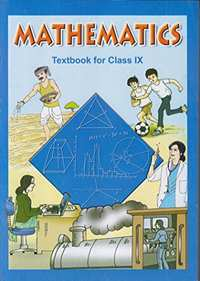Chapter 10: Circles

Ex. 10.10Ex. 10.20Ex. 10.30Ex. 10.40Ex. 10.50Ex. 10.60

Chapter 10: Circles Exercise 10.10 solutions [Page 171]

Ex. 10.10 | Q 1.1 | Page 171

Fill in the blanks:

The centre of a circle lies in ____________ of the circle.

• exterior

• interior

Ex. 10.10 | Q 1.2 | Page 171

Fill in the blanks:

A point, whose distance from the centre of a circle is greater than its radius lies in __________ of the circle. (exterior/ interior)

Ex. 10.10 | Q 1.3 | Page 171

Fill in the blanks:

The longest chord of a circle is a __________ of the circle.

Ex. 10.10 | Q 1.4 | Page 171

Fill in the blanks:

An arc is a __________ when its ends are the ends of a diameter.

Ex. 10.10 | Q 1.5 | Page 171

Fill in the blanks:

Segment of a circle is the region between an arc and __________ of the circle.

Ex. 10.10 | Q 1.6 | Page 171

Fill in the blanks:

A circle divides the plane, on which it lies, in __________ parts.

Ex. 10.10 | Q 2.1 | Page 171

Line segment joining the centre to any point on the circle is a radius of the circle.

• True

• False

Ex. 10.10 | Q 2.2 | Page 171

A circle has only finite number of equal chords.

• True

• False

Ex. 10.10 | Q 2.3 | Page 171

If a circle is divided into three equal arcs, each is a major arc.

• True

• False

Ex. 10.10 | Q 2.4 | Page 171

A chord of a circle, which is twice as long as its radius, is a diameter of the circle.

• True

• False

Ex. 10.10 | Q 2.5 | Page 171

Sector is the region between the chord and its corresponding arc.

• True

• False

Ex. 10.10 | Q 2.6 | Page 171

A circle is a plane figure.

• True

• False

Chapter 10: Circles Exercise 10.20 solutions [Page 173]

Ex. 10.20 | Q 1 | Page 173

Recall that two circles are congruent if they have the same radii. Prove that equal chords of congruent circles subtend equal angles at their centres.

Ex. 10.20 | Q 2 | Page 173

Prove that if chords of congruent circles subtend equal angles at their centres, then the chords are equal.

Chapter 10: Circles Exercise 10.30 solutions [Page 176]

Ex. 10.30 | Q 1 | Page 176

Draw different pairs of circles. How many points does each pair have in common? What is the maximum number of common points?

Ex. 10.30 | Q 2 | Page 176

Suppose you are given a circle. Give a construction to find its centre.

Ex. 10.30 | Q 3 | Page 176

If two circles intersect at two points, prove that their centres lie on the perpendicular bisector of the common chord.

Chapter 10: Circles Exercise 10.40 solutions [Page 179]

Ex. 10.40 | Q 1 | Page 179

Two circles of radii 5 cm and 3 cm intersect at two points and the distance between their centres is 4 cm. Find the length of the common chord.

Ex. 10.40 | Q 2 | Page 179

If two equal chords of a circle intersect within the circle, prove that the segments of one chord are equal to corresponding segments of the other chord.

Ex. 10.40 | Q 3 | Page 179

If two equal chords of a circle intersect within the circle, prove that the line joining the point of intersection to the centre makes equal angles with the chords.

Ex. 10.40 | Q 4 | Page 179

If a line intersects two concentric circles (circles with the same centre) with centre O at A, B, C and D, prove that AB = CD (See given figure)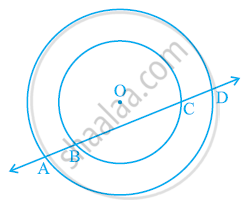Ex. 10.40 | Q 5 | Page 179

Three girls Reshma, Salma and Mandip are playing a game by standing on a circle of radius 5m drawn in a park. Reshma throws a ball to Salma, Salma to Mandip, Mandip to Reshma. If the distance between Reshma and Salma and between Salma and Mandip is 6m each, what is the distance between Reshma and Mandip?

Ex. 10.40 | Q 6 | Page 179

A circular park of radius 20 m is situated in a colony. Three boys Ankur, Syed and David are sitting at equal distance on its boundary each having a toy telephone in his hands to talk each other. Find the length of the string of each phone.

Chapter 10: Circles Exercise 10.50 solutions [Pages 184 - 186]

Ex. 10.50 | Q 1 | Page 184

In the given figure, A, B and C are three points on a circle with centre O such that ∠BOC = 30° and ∠AOB = 60°. If D is a point on the circle other than the arc ABC, find ∠ADC.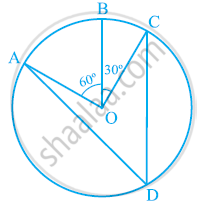Ex. 10.50 | Q 2 | Page 185

A chord of a circle is equal to the radius of the circle. Find the angle subtended by the chord at a point on the minor arc and also at a point on the major arc.

Ex. 10.50 | Q 3 | Page 185

In the given figure, ∠PQR = 100°, where P, Q and R are points on a circle with centre O. Find ∠OPR.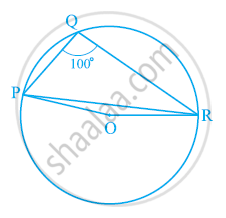Ex. 10.50 | Q 4 | Page 185

In the given figure, ∠ABC = 69°, ∠ACB = 31°, find ∠BDC.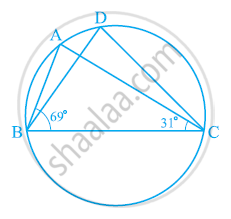Ex. 10.50 | Q 5 | Page 185

In the given figure, A, B, C and D are four points on a circle. AC and BD intersect at a point E such that ∠BEC = 130° and ∠ECD = 20°. Find ∠BAC.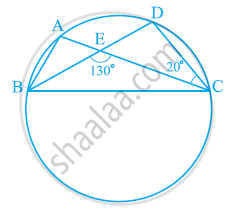Ex. 10.50 | Q 6 | Page 185

ABCD is a cyclic quadrilateral whose diagonals intersect at a point E. If ∠DBC = 70°, ∠BAC is 30°, find ∠BCD. Further, if AB = BC, find ∠ECD.

Ex. 10.50 | Q 7 | Page 185

If diagonals of a cyclic quadrilateral are diameters of the circle through the vertices of the quadrilateral, prove that it is a rectangle.

Ex. 10.50 | Q 8 | Page 185

If the non-parallel sides of a trapezium are equal, prove that it is cyclic.

Ex. 10.50 | Q 9 | Page 186

Two circles intersect at two points B and C. Through B, two line segments ABD and PBQ are drawn to intersect the circles at A, D and P, Q respectively (see the given figure). Prove that ∠ACP = ∠QCD.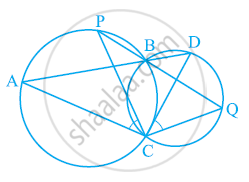Ex. 10.50 | Q 10 | Page 186

If circles are drawn taking two sides of a triangle as diameters, prove that the point of intersection of these circles lie on the third side.

Ex. 10.50 | Q 11 | Page 186

ABC and ADC are two right triangles with common hypotenuse AC. Prove that ∠CAD = ∠CBD.

Ex. 10.50 | Q 12 | Page 186

Prove that a cyclic parallelogram is a rectangle.

Chapter 10: Circles Exercise 10.60 solutions [Pages 186 - 187]

Ex. 10.60 | Q 1 | Page 186

Prove that the line of centres of two intersecting circles subtends equal angles at the two points of intersection.

Ex. 10.60 | Q 2 | Page 186

Two chords AB and CD of lengths 5 cm 11cm respectively of a circle are parallel to each other and are on opposite sides of its centre. If the distance between AB and CD is 6 cm, find the radius of the circle.

Ex. 10.60 | Q 3 | Page 187

The lengths of two parallel chords of a circle are 6 cm and 8 cm. If the smaller chord is at distance 4 cm from the centre, what is the distance of the other chord from the centre?

Ex. 10.60 | Q 4 | Page 186

Let the vertex of an angle ABC be located outside a circle and let the sides of the angle intersect equal chords AD and CE with the circle. Prove that ∠ABC is equal to half the difference of the angles subtended by the chords AC and DE at the centre.

Ex. 10.60 | Q 5 | Page 186

Prove that the circle drawn with any side of a rhombus as diameter passes through the point of intersection of its diagonals.

Ex. 10.60 | Q 6 | Page 186

ABCD is a parallelogram. The circle through A, B and C intersect CD (produced if necessary) at E. Prove that AE = AD.

Ex. 10.60 | Q 7 | Page 186

AC and BD are chords of a circle which bisect each other. Prove that (i) AC and BD are diameters; (ii) ABCD is a rectangle.

Ex. 10.60 | Q 8 | Page 187

Bisectors of angles A, B and C of a triangle ABC intersect its circumcircle at D, E and F respectively. Prove that the angles of the triangle DEF are 90^@-1/2A, 90^@-1/2B" and "90^@-1/2C

Ex. 10.60 | Q 9 | Page 186

Two congruent circles intersect each other at points A and B. Through A any line segment PAQ is drawn so that P, Q lie on the two circles. Prove that BP = BQ.

Ex. 10.60 | Q 10 | Page 186

In any triangle ABC, if the angle bisector of ∠A and perpendicular bisector of BC intersect, prove that they intersect on the circumcircle of the triangle ABC.

Chapter 10: Circles

Ex. 10.10Ex. 10.20Ex. 10.30Ex. 10.40Ex. 10.50Ex. 10.60

NCERT Mathematics Class 9NCERT solutions for Class 9 Mathematics chapter 10 - Circles

NCERT solutions for Class 9 Maths chapter 10 (Circles) include all questions with solution and detail explanation. This will clear students doubts about any question and improve application skills while preparing for board exams. The detailed, step-by-step solutions will help you understand the concepts better and clear your confusions, if any. Shaalaa.com has the CBSE Mathematics Textbook for Class 9 solutions in a manner that help students grasp basic concepts better and faster.

Further, we at shaalaa.com are providing such solutions so that students can prepare for written exams. NCERT textbook solutions can be a core help for self-study and acts as a perfect self-help guidance for students.

Concepts covered in Class 9 Mathematics chapter 10 Circles are Cyclic Quadrilaterals, Angle Subtended by an Arc of a Circle, Equal Chords and Their Distances from the Centre, Circle Through Three Points, Perpendicular from the Centre to a Chord, Angle Subtended by a Chord at a Point, Circles and Its Related Terms.

Using NCERT Class 9 solutions Circles exercise by students are an easy way to prepare for the exams, as they involve solutions arranged chapter-wise also page wise. The questions involved in NCERT Solutions are important questions that can be asked in the final exam. Maximum students of CBSE Class 9 prefer NCERT Textbook Solutions to score more in exam.

Get the free view of chapter 10 Circles Class 9 extra questions for Maths and can use shaalaa.com to keep it handy for your exam preparation

S